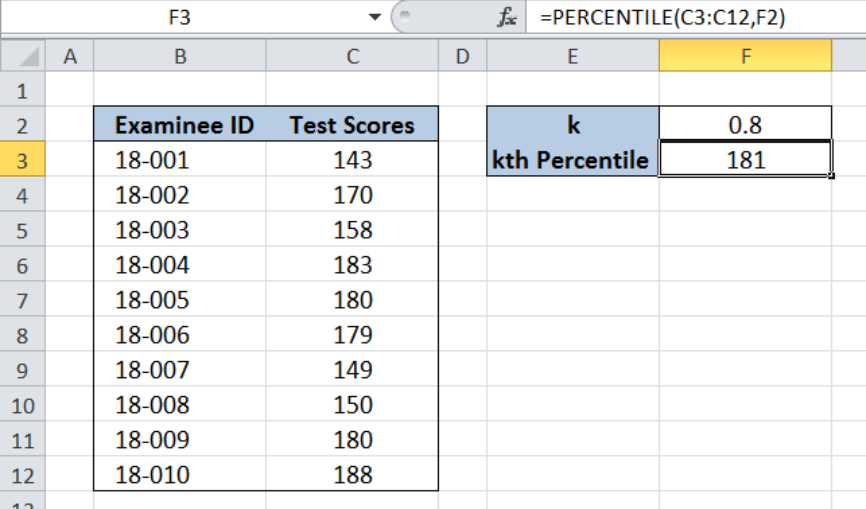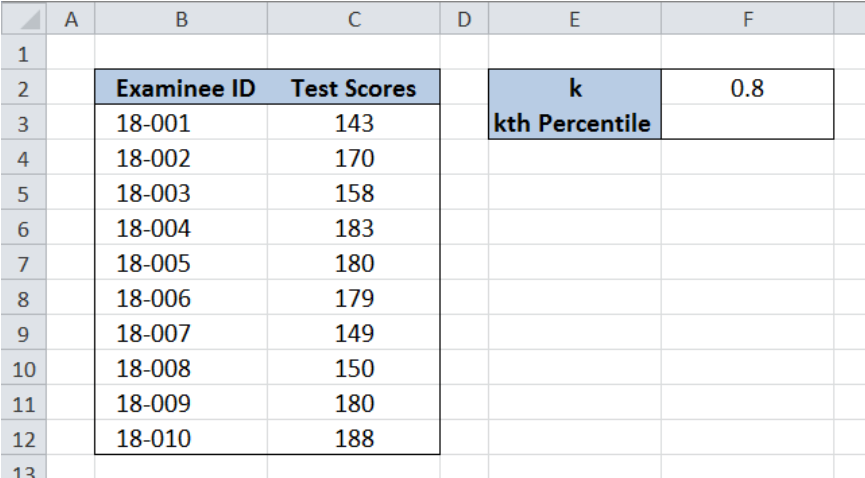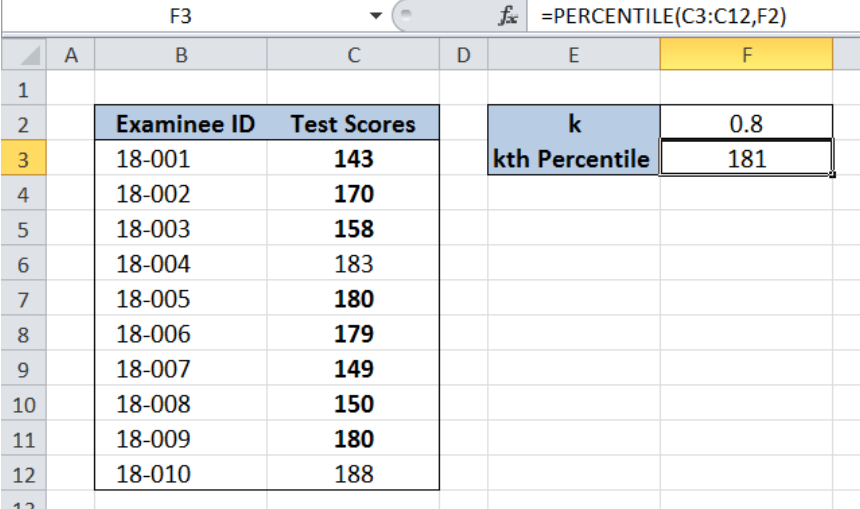Get instant live expert help with Excel or Google Sheets“My Excelchat expert helped me in less than 20 minutes, saving me what would have been 5 hours of work!”

#### Post your problem and you’ll get Expert help in seconds.

Your message must be at least 40 characters
Our professional Expert are available now. Your privacy is guaranteed.

# Excel PERCENTILE Function

Percentile is the value below which a percentage of our data falls.  Percentiles describe characteristics of distributions.  This step by step tutorial will assist all levels of Excel users in using the PERCENTILE function.Figure 1. Final result: Determine the 80th percentile

## Syntax of the PERCENTILE Function

PERCENTILE calculates the “kth percentile” for a given data set

### Syntax

`=PERCENTILE(array,k)`

where

• array – a range of values or data set whose “kth” percentile we want to determine
• k – the percentile value that ranges from 0 to 1

## Setting up the Data

Our table consists of two columns: Examinee ID (column B) and Test Scores (column C).  In cell F2, we enter 0.8 to calculate the 80th percentile of the Test Scores. The result for the 80th percentile will be recorded in cell F3.Figure 2. Sample data to determine the 80th percentile

## Determine 80th percentile

In order to determine the 80th percentile, we follow these steps:

Step 1. Select cell F3

Step 2. Enter the formula: `=PERCENTILE(C3:C12,F2)`

Step 3. Press Enter

The array is the range C3:C12, which contains the Test Scores.  The value for k is 0.8, which refers to the 80th percentile.  As a result, the PERCENTILE function returns 181, which means that 80% of the examinees have scores below 181.Figure 3.  Calculating the 80th percentile

Most of the time, the problem you will need to solve will be more complex than a simple application of a formula or function. If you want to save hours of research and frustration, try our live Excelchat service! Our Excel Experts are available 24/7 to answer any Excel question you may have. We guarantee a connection within 30 seconds and a customized solution within 20 minutes.

### Did this post not answer your question? Get a solution from connecting with the expert.Another blog reader asked this question today on Excelchat:## Subscribe to Excelchat.coAnother blog reader asked this question today on Excelchat: